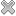# Courses / Module

Toggle Print

##PHYSICS FOR ENGINEERS 1

Module code: EE104FZ
Credits: 5
Semester: 1
Department: INTERNATIONAL ENGINEERING COLLEGE
International:Overview

The aims of this module are: * To introduce students to the physics of mechanics and heat, and to tutor them in the solution of problems in these two areas. * To emphasize problem-solving: setting up diagrams of situations, setting up equilibrium equations, and solving these equations Indicative Syllabus: _Mechanics: (approx 18 lectures) *Introduction and mathematical concepts; Vectors and vector components *Linear Motion with Constant Acceleration; Projectile Motion *Relative Velocity; Newton’s Laws of Motion and Engineering Applications *Free body Diagrams, Equilibrium Equations *Circular Motion, Centripetal Forces *Work and Energy, Conservative and Non-conservative forces *Impulse and Momentum, Law of Conservation of Momentum, Elastic and non-elastic collisions _Heat: (approx 6 lectures) *Temperature and heat, Thermal Expansion, Heat Capacity, Latent Heat *The transfer of heat: Convection, Conduction, RadiationLearning OutcomesTeaching & Learning methodsAssessmentRepeat optionsTimetable